# 深入理解C++指针

1.        #include<iostream>

2.        using namespace std;

3.        void main()

4.        {

5.                 //字符指针指向一个字符串

6.                 cout << "字符指针测试部分："<< endl;

7.                 char*p = "csdn";//定义一个字符型的指针，并用一个字符串初始化

8.                 cout << p << endl;//输出p所指向的字符串的值，C++中默认输出整个字符串

9.                 cout << (void*)p << endl;//输出指针p指向的地址

10.             cout << *p << endl;//输出p指向地址所存储的字符

11.             cout << (void*)&(*p) << endl;//输出该字符的地址

12.             cout << *(++p) << endl;//输出p指针指向的地址的下一个地址的存储的值

13.             cout << (void*)& *(++p) << endl;//输出该字符的地址

14.

15.             cout << "字符数组测试部分："<< endl;

16.             //字符数组存储一个字符串

17.             char s[] = "csdn";//定义一个字符型数组，并用一个字符串初始化

18.             cout << s << endl;//输出s所指向的字符串的值，C++中默认输出整个字符串

19.             cout << (void*)s << endl;//输出该字符数组的首地址

20.             cout << *s << endl;//输出s所代表地址的存储的值

21.             cout << &s << endl;//输出s所代表的地址

22.             cout << s << endl;//输出s的下一个地址的存储值

23.             cout << (void*)&s << endl;//输出该字符的地址

24.             }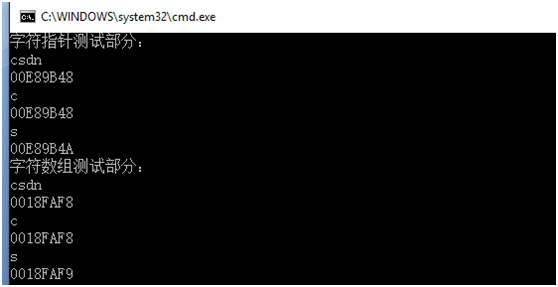1.        #include<iostream>

2.        using namespace std;

3.        void main()

4.        {

5.                 char*p = "csdn";//定义一个字符型的指针，并用一个字符串初始化

6.                 cout << (void*)p << endl;//输出指针p指向的地址

7.                 cout << &p << endl;//输出存储指针变量p的地址空间

8.                 cout << *(++p) << endl;//输出p指针指向的地址的下一个地址的存储的值

9.                 cout << (void*)& *(++p) << endl;//输出该字符的地址

10.    }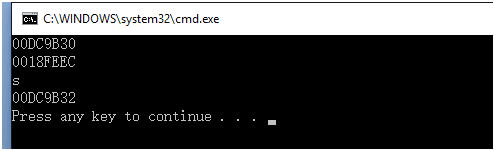1.        #include<iostream>

2.        using namespace std;

3.        void main()

4.        {

5.                 char s[] = "csdn";//定义一个字符型数组，并用一个字符串初始化

6.                 cout << (void*)s << endl;//输出指针p指向的地址

7.                 cout << &s << endl;//输出存储指针变量p的地址空间

8.                 cout << *(++s) << endl;//输出p指针指向的地址的下一个地址的存储的值

9.                 cout << (void*)& *(++s) << endl;//输出该字符的地址

10.    }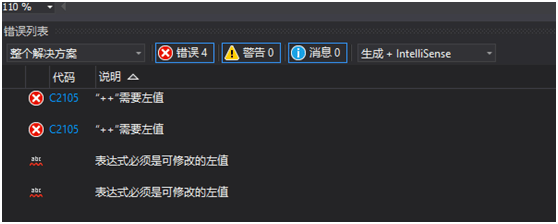#include<iostream>

1.          using namespace std;

2.          void main()

3.          {

4.                   char s[] = "csdn";//定义一个字符型数组，并用一个字符串初始化

5.                   cout << (void*)s << endl;//输出指针p指向的地址

6.                   cout << &s << endl;//输出存储指针变量p的地址空间

7.          }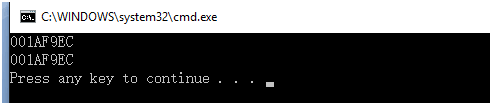1.        #include<iostream>

2.        using namespace std;

3.        void main()

4.        {

5.                 char s[] = "csdn";//定义一个字符型数组，并用一个字符串初始化

6.                 *s = 'A';//修改字符串第一地址的字符值

7.                 cout << s << endl;//重新输出字符串

8.                 char*p = "csdn";//定义一个字符型的指针，并用一个字符串初始化

9.                 *p = 'A';// 修改字符串第一地址的字符值

10.             cout << p << endl; //重新输出字符串

11.    }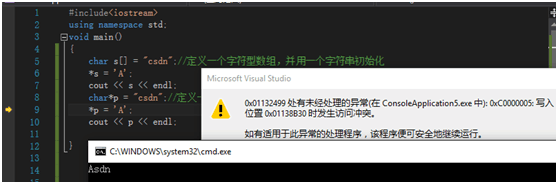1.        #include<iostream>

2.        using namespace std;

3.        void main()

4.        {

5.                 char s[] = "csdn";//定义一个字符型数组，并用一个字符串初始化

6.                 cout << &s << endl;//输出s绑定的地址值

7.                 char*p = s;//定义一个字符型的指针，并把s绑定的值赋给p

8.                 cout << (void*)p << endl;//输出指针变量p存储的值

9.                 *p = 'A';//利用指针变量修改字符串的首字母

10.             cout << p << endl;//输出修改后的字符串

11.    }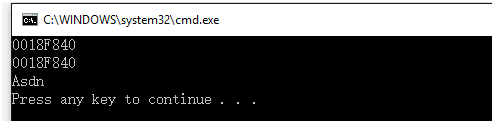03-293万+
04-081万+
09-241万+
11-272744
09-01157
08-18
11-20
06-12
12-31
01-11
11-11

### “相关推荐”对你有帮助么？

•非常没帮助
•没帮助
•一般
•有帮助
•非常有帮助被折叠的  条评论 为什么被折叠?到【灌水乐园】发言Jeff_Scott

¥2 ¥4 ¥6 ¥10 ¥20余额支付 (余额：-- )扫码支付获取中扫码支付点击重新获取扫码支付1.余额是钱包充值的虚拟货币，按照1:1的比例进行支付金额的抵扣。
2.余额无法直接购买下载，可以购买VIP、C币套餐、付费专栏及课程。余额充值